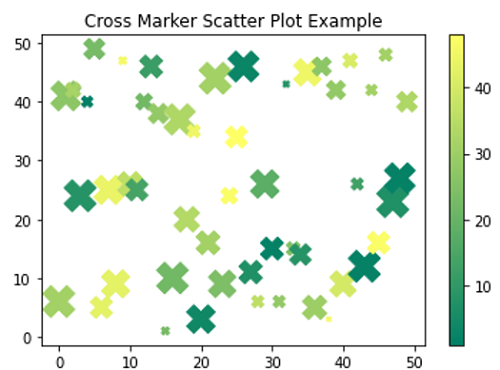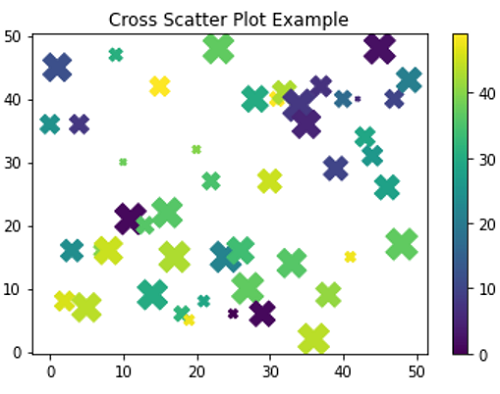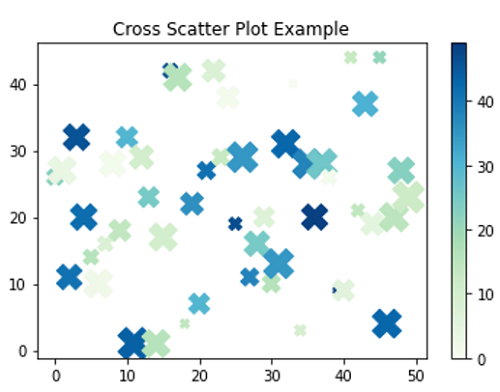# Python | Cross(X) Scatter Marker in Matplotlib

In this tutorial, we are going to learn how to use cross (X) shape scatter marker in scatter plot using matplotlib in Python?
Submitted by Anuj Singh, on August 16, 2020

There are few markers that can be used everywhere such as circular or square markers. But, matplotlib has some other inbuilt defined markers such as cross(X) shape marker which is used in marking plots.

In matplotlib.pyplot command marker='4' for our desired marker style and the following figure illustrates the example of the same.

Illustrations:## Python code for cross(x) scatter marker in matplotlib

```import numpy as np
import matplotlib.pyplot as plt

# Example 1
x = np.arange(50)
y = np.random.randint(0,50,50)
ss = np.random.randint(0,50,50)
c = np.random.randint(0,50,50)

plt.figure()
plt.scatter(x,y, s=ss*10, c=c, marker='X', cmap='summer')
plt.title('Cross Marker Scatter Plot Example')
plt.colorbar()

# Example 2
x = np.arange(50)
y = np.random.randint(0,50,50)
ss = np.random.randint(0,50,50)
c = np.random.randint(0,50,50)

plt.figure()
plt.scatter(x,y, s=ss*10, c=c, marker='X')
plt.title('Cross Scatter Plot Example')
plt.colorbar()

# Example 3
x = np.arange(50)
y = np.random.randint(0,50,50)
ss = np.random.randint(0,50,50)
c = np.random.randint(0,50,50)

plt.figure()
plt.scatter(x,y, s=ss*10, c=c, marker='X', cmap='GnBu')
plt.title('Cross Scatter Plot Example')
plt.colorbar()
```

Output:

```Output is as Figure
```

Languages: » C » C++ » C++ STL » Java » Data Structure » C#.Net » Android » Kotlin » SQL
Web Technologies: » PHP » Python » JavaScript » CSS » Ajax » Node.js » Web programming/HTML
Solved programs: » C » C++ » DS » Java » C#
Aptitude que. & ans.: » C » C++ » Java » DBMS
Interview que. & ans.: » C » Embedded C » Java » SEO » HR
CS Subjects: » CS Basics » O.S. » Networks » DBMS » Embedded Systems » Cloud Computing
» Machine learning » CS Organizations » Linux » DOS
More: » Articles » Puzzles » News/Updates